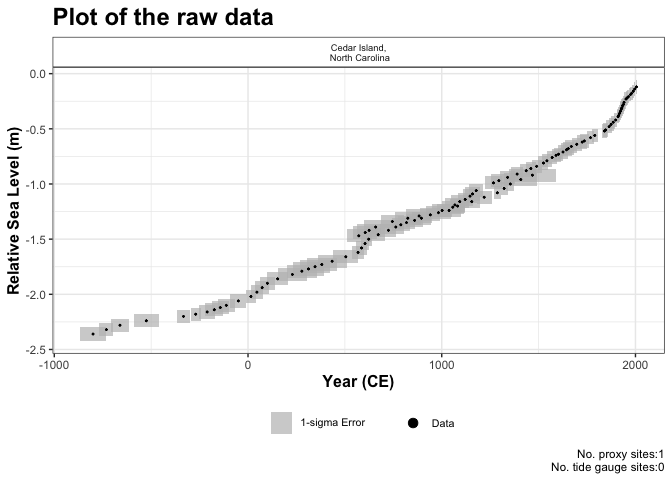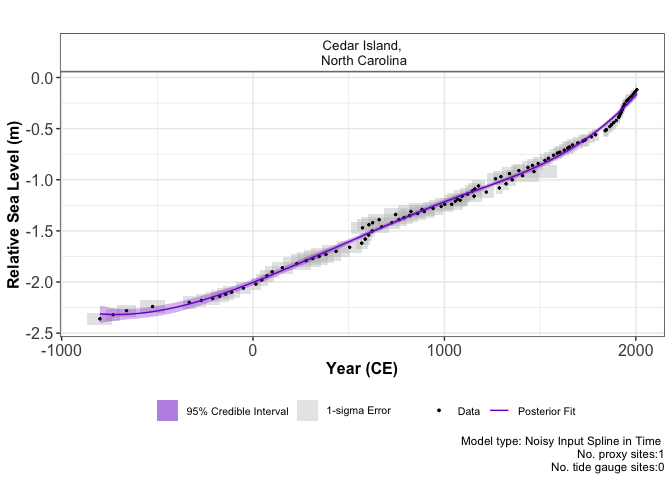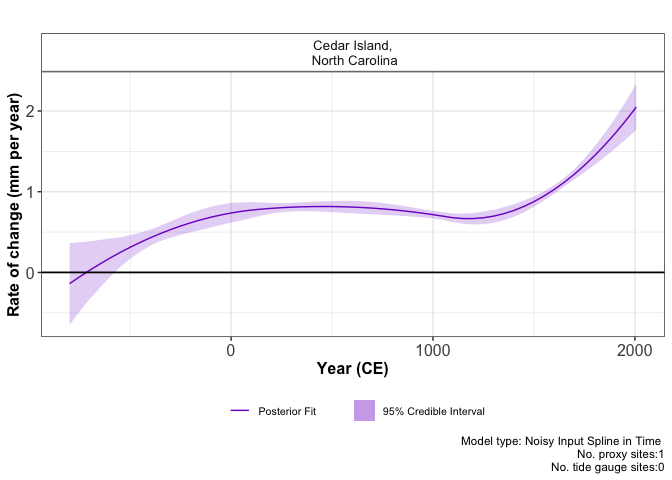# reslr: quick start guide

## Step 1: install reslr

Use:

# Not on CRAN yet
#install.packages("reslr")
#devtools::install_github("maeveupton/reslr")
install_github("maeveupton/reslr")

then,

library(reslr)

Note: The JAGS software is a requirement for this instruction sheet and refer back to main vignettes for more information.

## Step 2: load in the data into reslr

There is a large example dataset included in the reslr package called NAACproxydata. In this example, we demonstrate how to include proxy record data which is stored in a csv file. This csv file of data can be found in the package and the readr function reads the csv file:

path_to_data <- system.file("extdata", "one_data_site_ex.csv", package = "reslr")
example_one_datasite <- read.csv(path_to_data)

Using the reslr_load function to read in the data into the reslr package:

example_one_site_input <- reslr_load(
data = example_one_datasite)

## Step 3: plot the data

plot(
x = example_one_site_input,
title = "Plot of the raw data",
xlab = "Year (CE)",
ylab = "Relative Sea Level (m)",
plot_tide_gauges = FALSE,
plot_caption = TRUE
)## Step 4: Run your statistical model and check convergence

Select your modelling technique from the modelling options available:

Statistical Model Model Information model_type code
Errors in variables simple linear regression A straight line of best fit taking account of any age and measurement errors in the RSL values using the method of Cahill et al (2015). Use for single proxy site. “eiv_slr_t”
Errors in variables change point model An extension of the linear regression modelling process. It uses piece-wise linear sections and estimates where/when trend changes occur in the data (Cahill et al. 2015). “eiv_cp_t”
Errors in variables integrated Gaussian Process A non linear fit that utilities a Gaussian process prior on the rate of sea-level change that is then integrated (Cahill et al. 2015). “eiv_igp_t”
Noisy Input spline in time A non-linear fit using regression splines using the method of Upton et al (2023). “ni_spline_t”
Noisy Input spline in space and time A non-linear fit for a set of sites across a region using the method of Upton et al (2023). “ni_spline_st”
Noisy Input Generalised Additive model for the decomposition of the RSL signal A non-linear fit for a set of sites across a region and provides a decomposition of the signal into regional, local-linear (commonly GIA) and local non-linear components. Again this full model is as described in Upton et al (2023). “ni_gam_decomp”

For this example, it is a single site and we are interested in how it varies over time select the Noisy Input spline in time. If it was multiple sites, we recommend using a spatial temporal model, i.e. Noisy Input spline in space and time, or for decomposing the signal, i.e. Noisy Input Generalised Additive model.

Once the model is chosen use the reslr_mcmc function to run it:

res_one_site_example <- reslr_mcmc(
input_data = example_one_site_input,
model_type = "ni_spline_t",
CI = 0.95
)

The convergence of the algorithm is examined and he parameter estimates from the model can be investigated using the following:

summary(res_one_site_example)
#> No convergence issues detected.
#> # A tibble: 2 × 7
#>   variable      mean      sd     mad       q5    q95  rhat
#>   <chr>        <num>   <num>   <num>    <num>  <num> <num>
#> 1 sigma_beta 2.09    0.685   0.529   1.29     3.33    1.00
#> 2 sigma_y    0.00624 0.00489 0.00460 0.000447 0.0156  1.00

## Step 5: Plot the results

The model fit results can be visualised using the following function:

plot(res_one_site_example,
xlab = "Year (CE)",
ylab = "Relative Sea Level (m)",
plot_type = "model_fit_plot"
)For the rate of change plot use:

plot(res_one_site_example,
plot_type = "rate_plot"
)To examine the data creating these plots the user types the following:

output_dataframes <- res_one_site_example\$output_dataframes
#>   Longitude Latitude                       SiteName data_type_id  Age      pred
#> 1    -76.38   34.971 Cedar Island,\n North Carolina  ProxyRecord -800 -2.312202
#> 2    -76.38   34.971 Cedar Island,\n North Carolina  ProxyRecord -750 -2.316994
#> 3    -76.38   34.971 Cedar Island,\n North Carolina  ProxyRecord -700 -2.317622
#> 4    -76.38   34.971 Cedar Island,\n North Carolina  ProxyRecord -650 -2.314291
#> 5    -76.38   34.971 Cedar Island,\n North Carolina  ProxyRecord -600 -2.307204
#> 6    -76.38   34.971 Cedar Island,\n North Carolina  ProxyRecord -550 -2.296567
#>         upr       lwr   rate_pred    rate_upr  rate_lwr  CI
#> 1 -2.399767 -2.229546 -0.13882006 -0.65081467 0.3625245 95%
#> 2 -2.386640 -2.250235 -0.05351566 -0.48182156 0.3739311 95%
#> 3 -2.374637 -2.261549  0.02770941 -0.32980947 0.3860676 95%
#> 4 -2.364049 -2.262925  0.10485494 -0.19083120 0.4022410 95%
#> 5 -2.356266 -2.258588  0.17792093 -0.05568056 0.4203347 95%
#> 6 -2.345550 -2.247646  0.24690738  0.06597339 0.4350460 95%

To examine the additional options in the reslr package, see the main vignette.